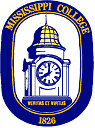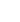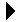# Shuffle a listCS 231 Lecture ExamplesDownload```; ; Move the nth member of the list to the front. If n is too large, the ; last member is moved. If n is too small, no change. Items are numbered ; from zero. The empy list is returned unchanged. (defun getnth (n lst) (cond ((null lst) lst) ; List empty. Return it. ((< n 0) lst) ; n is too small. Return list. ((null (cdr lst)) lst) ; Reached last member of list. Return list. ((= n 0) lst) ; Right thing already at front. (t ; The nth member of lst is the n-1st member of (cdr lst). ; Move the n-1 st member of (cdr lst) to its front, then ; construct the final result. (let* ((moverest (getnth (- n 1) (cdr lst)))) (cons (car moverest) (cons (car lst) (cdr moverest)))) ) ) ) ; ; Shuffle the list. (defun shuffle (lst) (cond ((null lst) lst) (t (let* ( (llen (length lst)) (ranfront (getnth (random llen) lst)) ) (cons (car ranfront) (shuffle (cdr ranfront))) ) ) ) ) ```